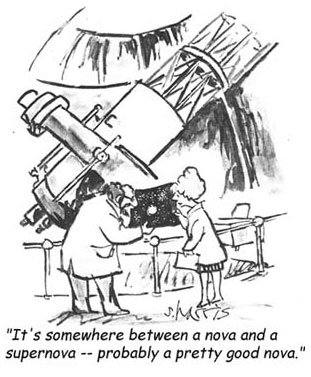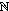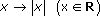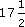Approximations are most useful when it comes to making rough estimates –
Author(s): The Open University

## Activity 5

Round a measurement of 1.059 metres:

• (a) to the nearest whole number of metres;

• (b) to two decimal places;

Author(s): The Open University

The blouse in the figure above was £19 and you may well have thought of it as roughly £20. In this case you would be rounding to the nearest ten (pounds).

The rule for rounding to the nearest hundred can be adapted easily to rounding to the nearest ten. Instead of looking at the tens digit look at the units digit.

So £23 is rounded down (to £20) and £36 is rounded up (to £40).

Author(s): The Open University

You will probably think to yourself that the coat shown costs about £300. £290 is considerably closer to £300 than it is to £200, so £300 is a reasonable approximation. In this case, 290 has been rounded up to 300. Similarly, 208 would be rounded down to 200 because it is closer to 200 than it is to 300. Both numbers have been rounded to the nearest hundred pounds.

When rounding to the nearest hundred, anything below fifty rounds down. So 248 rounds to 200. Anything o
Author(s): The Open University

In OpenLearn course M208_5 Mathematical language you met the sets

•= {1, 2, 3, …}, the natural numbers;

• [Image_Link]https://www.open.edu/
Author(s):
The Open University## Study another free course

There are more than 800 courses on OpenLearn for you to
Author(s): The Open University

## Question 1

Calculate all the angles at the centres of these objects.

The modulus function provides us with a measure of distance that turns the set of complex numbers into a metric space in much the same way as does the modulus function defined on R. From the point of view of analysis the importance of this is that we can talk of the closeness of two complex numbers. We can then define the limit of a sequence of complex numbers in a way which is almost identical to the definition of the limit of a real sequence. Another analogue of real analysis arises
Author(s): The Open University

You will no doubt recall that in real analysis extensive use is made of the modulus function. It gives us a way of measuring the “closeness” of two numbers, which we exploit in writing expressi
Author(s): The Open University

## SAQ 13

Find |z| and Arg z in each of the following cases.

1. [Image_Link]https://www.open.edu/openlearn/ocw/pluginfil
Author(s): The Open University

In this section we have seen a correspondence between complex numbers and points in the plane using Cartesian coordinates; the real part of the complex number is represented on the real axis (“horizontal”) and the imaginary part on the imaginary axis (“vertical”). We can also use polar coordinates (r,θ) in which case r, the modulus of a non-zero complex number z is positive and θ is an argument of z, defined only to within an additive intege
Author(s): The Open University

In this section we shall define the complex number system as the set R × R (the Cartesian product of the set of reals, R, with itself) with suitable addition and multiplication operations. We shall define the real and imaginary parts of a complex number and compare the properties of the complex number system with those of the real number system, particularly from the point of view of analysis.

Author(s): The Open University## Study another free course

There are more than 800 courses on OpenLearn for you to
Author(s): The Open University

In Section 5 we show how functions may be used to sketch curves in the plane, even when these curves are not necessarily the graphs of functions.

Click the link below to open Section 5 (8 pages, 151KB).

Section 5

Author(s): The Open University

In Section 3 we consider how to sketch the graphs of more complicated functions, sometimes involving trigonometric functions. We look at graphs which are sums, quotients and composites of different functions, and at those which are defined by a different rule for different values of x.

Click the link below to open Section 3 (7 pages, 133KB).

In Section 2 we describe how the graphs of polynomial and rational functions may be sketched by analysing their behaviour – for example, by using techniques of calculus. We assume that you are familiar with basic calculus and that its use is valid. In particular, we assume that the graphs of the functions under consideration consist of smooth curves.

Click the link below to open Section 2 (16 pages, 200KB).

Author(s): The Open University

Our everyday experience of percentages includes percentage increases (like VAT at%, or a service charge of 15%) and percentage decreases (such as a discount of 15%).

For example, £8 plus
Author(s): The Open University

## Activity 3

A local supermarket sells a popular breakfast cereal in a ‘Large Pack’ and ‘New Extra Large Pack’. They are both being sold at ‘knock down’ prices. The large pack contains 450 g of cereal priced at �
Author(s): The Open University

Studying mammals: Food for thought
Who were our ancestors? How are apes and humans related? And where does the extinct Homo erectus fit into the puzzle? In this free course, Studying mammals: Food for thought, we will examine culture, tool use and social structure in both apes and humans to gain an understanding of where we come from and why we behave as we do. This is the tenth course in the Studying mammals series. Author(s): Creator not set

This course will facilitate your exploration of a viable way of life by identifying actions that individuals can take to reduce environmental impacts while sustaining community well-being. You will be required to develop a worked example of how to go about doing this based on your own experience. In particular, you will explore how identifying a balance between reducing environmental impacts and sustaining community well-being should be an ongoing Author(s): The Open University